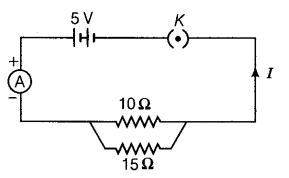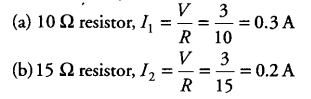# Study the following circuit and answer the questions that follows:

Study the following circuit and answer the questions that follows:(i) State the type of combination of two resistors in the circuit.
(ii) How much current is flowing through
(a) 10 ohms and
(b) 15 ohms resistor?
(iii) What is the ammeter reading?

(i) Two resistors are in parallel combination.
(ii) Current through(iii) Ammeter reading = Total current flowing through the circuit = 03 + 0.2 = 0.5 A